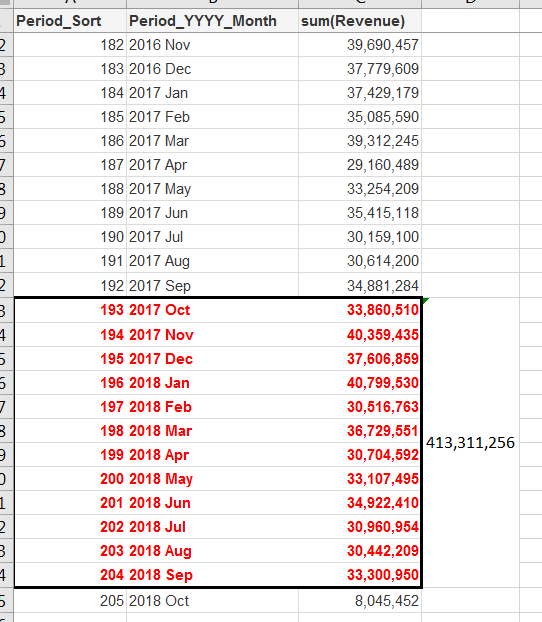New to QlikView

Discussion board where members can get started with QlikView.

Announcements
Contributor

Rolling 12 months calc, not showing correct figures

Hi all, hope you're having a good day.

I am hoping, you can solve my head scratching query, around a rolling 12 month calculation not working.

As per my attached qvd, there are two tables.

First one showing, 12 month totals per month.

The second, showing the individual months total

So if you added up 2018 Oct to 2017 Nov = 387,496,198, which you'll see from the first table is 100% correct.

If you do the next one being, 2018 Sep to 2017 Oct = 413,311,256 which then doesnt equal the first table of 413,307,617.

So i'm a little confused, why this is not showing the correct total for the 12 months total?!

Any help, would be greatly appreciated.

Tags (1)
1 Solution

Accepted SolutionsMVP

Re: Rolling 12 months calc, not showing correct figures

The problem was that your inner set analysis check against Client_Name also included set analysis to only look at >Max_Period - 11 <Max_Period. So, the limits were only checked for those revenues which fell within that date range. So, essentially, any customer which was in the limit but didn't have any sales within the date range was excluded. I removed that bit of the set analysis and boom you got what you needed.

Does that make sense?

19 RepliesMVP & Luminary

Re: Rolling 12 months calc, not showing correct figures

You need to sort the virtual table you create in the aggr function by the period/month. Try

sum(aggr(rangesum(above(sum({<Period_Sort>} Revenue),0,12)),(Period_Sort,(NUMERIC, ASCENDING))))

* Avg({<[Period_Sort]={">=\$(=max([Period_Sort])-11)<=\$(=max([Period_Sort]))"},Month= ,Year_YYYY= ,Quarter_QQ= , Period_YYYY_Month=>} 1)

talk is cheap, supply exceeds demand
Contributor

Re: Rolling 12 months calc, not showing correct figures

Hi Gysbert, many thanks for your reply, it's very much appreciatedusing just the calc you stated above does work on it's own!

However, did you see within my expression i've got two variables working at client level for vMinLimit and vMaxLimit?

i thought putting the aggr at the beginning of the calc and the numeric ascending after the Revenue),0,12 would work but this doesn't seem to work? have i missed something obvious?

=RangeSum(Above(Sum({<[Period_Sort]=,Month= ,Year_YYYY= ,Quarter_QQ= , Period_YYYY_Month=,

Client_Name={"=round(Sum({<[Period_Sort]={"">=\$(=max([Period_Sort])-11)<=\$(=max([Period_Sort]))""},Month= ,Year_YYYY= ,Quarter_QQ= ,Period_YYYY_Month =>}Revenue))<=\$(vMinLimit)
or
round(Sum({<[Period_Sort]={"">=\$(=max([Period_Sort])-11)<=\$(=max([Period_Sort]))""},Month= ,Year_YYYY= ,Quarter_QQ= ,Period_YYYY_Month= >}Revenue))>=\$(vMaxLimit)"
}>} Revenue), 0, 12))

*
Avg({<[Period_Sort]={">=\$(=max([Period_Sort])-11)<=\$(=max([Period_Sort]))"},Month= ,Year_YYYY= ,Quarter_QQ= , Period_YYYY_Month=>} 1)MVP & Luminary

Re: Rolling 12 months calc, not showing correct figures

Yes, you're missing a sum(aggr(.... around your rangesum.

talk is cheap, supply exceeds demand
Contributor III

Re: Rolling 12 months calc, not showing correct figures

I think he's also missing the red bit in ,(Period_Sort,(NUMERIC, ASCENDING)))) as he said he's only adding the numeric ascending.

By the way, is this only available in Qlikview 12? I've been following this thread because it seems interesting but when I enter your expression I just get 'Error in expression: ')' expected'. Am using Qlikview 11.MVP

Re: Rolling 12 months calc, not showing correct figures

Yes, this is only available in QV12 and above versions of QlikView. Available in most versions of Qlik Sense.

The sortable Aggr function is finally here!

Contributor III

Thanks Sunny!

Highlighted
Contributor

Re: Rolling 12 months calc, not showing correct figures

Hi guys, many thanks for the replies, an interesting subject this,

I've attached the updated expression, which I think it should be based on your replies and the answer is still not what i expected.

2018 Sep is Showing 413,307,617 when it should be 413,311,256.

Can you see any glaring mistakes, in my code?

Many thanks for taking the timeMVP

Re: Rolling 12 months calc, not showing correct figures

There is a difference between the two tables that you have... Individual month totals is for all clients (because it uses Sum(Revenue) as expression) whereas Rolling 12 month totals only include Client_Name which meet this condition

Client_Name = {"=Round(Sum({<[Period_Sort]={"">=\$(=max([Period_Sort])-11)<=\$(=max([Period_Sort]))""}, Month, Year_YYYY, Quarter_QQ, Period_YYYY_Month>}Revenue)) <= \$(vMinLimit)

or

Round(Sum({<[Period_Sort]={"">=\$(=max([Period_Sort])-11)<=\$(=max([Period_Sort]))""}, Month, Year_YYYY, Quarter_QQ, Period_YYYY_Month>}Revenue)) >= \$(vMaxLimit)"}

If you add this condition to the individual month totals, the monthly numbers will change and you give you 413,307,617. If you think, that 413,311,256 is the right number, then remove the set analysis from your Rolling 12 month totals tableContributor

Re: Rolling 12 months calc, not showing correct figures

Hi Sunny,

Appreciate your views as alwaysThe only reason I put the individual months table in there, was to show the real time numbers each month, so you could add the 12 months together.

As you can see below from the individual table, this is where i've got the 413,311,256 from!

So surely by using the set analysis, why doesn't this just include the Client_Name which meets this condition highlighted?

Thanks